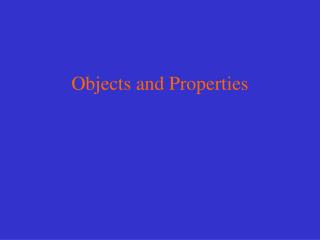DownloadDownload PresentationObjects and Properties

# Objects and Properties

Télécharger la présentation## Objects and Properties

- - - - - - - - - - - - - - - - - - - - - - - - - - - E N D - - - - - - - - - - - - - - - - - - - - - - - - - - -
##### Presentation Transcript

1. Objects and Properties

2. Objects : physical "things" in our environment • Properties of objects • those qualities that make an object what it is • Referent (reference) • how we view things due to our experiences • our window to the world • in physical science we need common referents(references) by which to study our physical universe

3. in science we need to eliminate vagueness in communication • we do this by having standard measurements with which to make comparisons between objects • A measurement consists of two parts: • numerical value: describes how big the measurement is • unit: tells us what the measurement is in; e.g., meters, kilograms, cubic centimeters, etc. • the unit also shows the type of measurement we are making; e.g., length, mass, volume, etc.

4. Making a measurement • A measurement consists of three activities: • choose a referent (reference), which will be your unit of measurement • compare the property you want to measure to the chosen reference (unit) • count the units (in the chosen reference)

5. Standard Units • in science a set of standard measuring units are used to ensure that measurements can be duplicated by others • Two major systems of standard units: • Metric System • Used throughout the world • Based on powers of 10 • English System • units which corresponded originally to parts of the human body

6. Many early units for measurement were originally based on the human body. Some of the units were later standardized by governments to become the basis of the English system of measurement.

7. Area (the size of a surface) can be described by two length measurements. Volume (the space an object occupies) can be described by three length measurements. Length, however, can be described only in terms of how it is measured, so it is called a fundamentalproperty. Area and volume are called derivedproperties.

8. Fundamental (Base) and Derived Properties • Fundamental (base) property • defined by itself, independent of other properties • Fundamental (base) properties are: • length, mass, time, temperature, electric current • Derived property • defined in terms of fundamental properties (depends on fundamental properties) • Examples: surface area, volume, speed (speed is distance divided by time), etc.

9. Metric system (MKS) - also called International System of Units (SI) Fundamental PropertiesBase UnitSymbol • Length meter m • Mass kilogram kg • Time second s • Temperature Kelvin K • Electric current ampere A

10. Base Units for the Metric System • Meter (m) • base unit of length: meter (m) 1m = 3.28 ft (foot is the base unit of length in the English system) • Kilogram (kg) • base unit of mass: kilogram (kg) • Mass represents the amount of matter (substance) contained in an object. The mass of an object is a measure of the inertia (resistance to movement) of that object. • Weight is the effect of gravity on an object. • The weight of an object changes with location, the mass of an object does not. • Second (s) • base unit of time: second (s) (also the base unit of time in the English system)

11. Each of the base units in the metric system can be modified with different prefixes which multiply out the base unit by some factor PrefixSymbolMeaning • Giga G 1,000,000,000 times the base unit • Mega M 1,000,000 times the base unit • Kilo k 1,000 times the base unit • Hecto h 100 times the base unit • Deka da 10 times the base unit • Deci d 0.1 of the base unit • Centi c 0.01 of the base unit • Milli m 0.001 of the base unit • Micro  0.000001 of the base unit • Nano n 0.000000001 of the base unit

12. The Scientific Method • Method for developing scientific knowledge • Steps: • Hypothesis: possible explanation of a phenomenon • Experiments: test the hypothesis • If the hypothesis fails, revise it and retest • If the hypothesis passes, more experiments for more testing and greater confidence • An accepted hypothesis may result in a scientific principle or in a scientific law • Scientific principle • An explanation concerned with a specific range of phenomena • Scientific law • Describes a more general and important phenomenon than a principle • Expressed: • in verbal form • by an equation • by a graph

13. Scientific Models and Theories • Model • simulation of a real object or phenomenon • used to study something that cannot be observed directly • physical model - can be seen and touched • mental model - exists in the mind and helps us understand concepts • equationmodel - describes the variables involved and their relationship • Theory • a broad, detailed explanation of a phenomenon • Hypothesis: statement before tests • Theory: has passed the test

14. Scientific Statements and Data • Must be testable (measurable) • Data (or measurements) must meet two criteria: • Reliability • everyone agrees to the meaning of the data (measurements) • others can replicate the measurements (or the experiments) with the same results • Precision • Repeatable and reproducible measurements that conform to standards